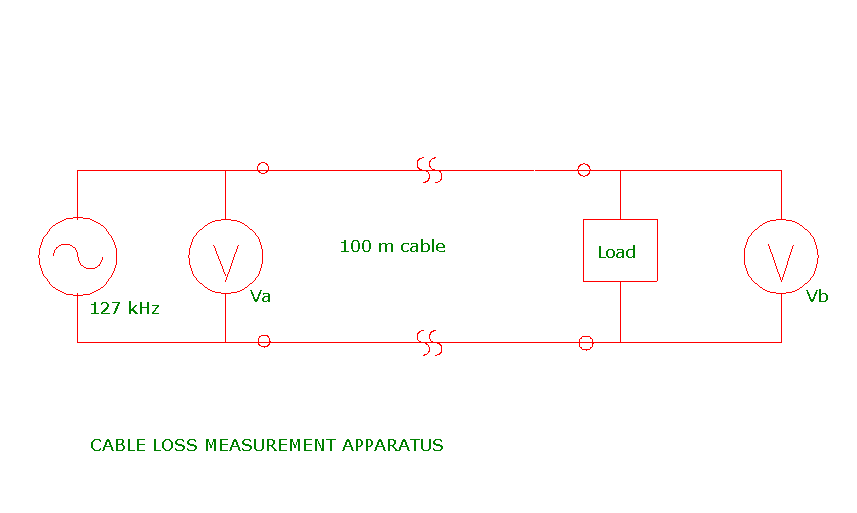# XYLENE POWER LTD.

## POWER LINE CARRIER LIGHTING CONTROL

### CABLE LOSS

#### 14 AWG 2 wire BX Cable (3/8" armour)

Data supplied by Systel indicates that at 100 kHz, the impedance of one Systel 2 lamp 70 watt ballast is 380 0hms inductive. Thus three such ballasts connected in parallel have an impedance of 380 / 3 = 127 ohms inductive. The corresponding inductance value is given by:
127 / (6.28 X 10^5) = 202 uH.
Hence three parallel connected ballasts have about the same impedance as two series connected 100 uH inductors.

At low frequencies the same three ballasts at full load draw 3 X 70 = 210 watts. The equivalent resistance of these three parallel connected ballasts is given by: R = V^2/P = (120)^2/210 = 68.6 ohms. This resistance can be approximately simulated by two series connected 36 ohm resistors.

We can conduct cable attenuation tests using a measured 100 m length of #14 2 wire (plus ground) 3/8 inch diameter armour cable.Thus, by using various terminations consisting of series/parallel combinations of 4 100 uH inductors and 4 36 ohm resistors, at 127 kHz we can prepare a table of the following form:
Termination------Equiv.No. of Ballasts------Transfer Ratio = Vb / Va
200 uH3
100 uH6
66.67 uH9
50 uH12
40 uH15
33.3 uH18.439
25 uH24.382
72 R3
36 R6
24 R9
18 R12
14.4 R15
12 R18
9 R24.234

Consider the case of the 9 ohm resistive termination. If we assume that for a 200 m cable the loss is (Vb / Va)^2 = (.234)^2 = .0547, and if we operate under the guideline that the cable loss fraction should be greater than 1/20 = .05, then we find that the maximum allowable cable length may be as short as 200 m for a fully loaded lighting circuit that contains a substantial fraction of incandescent lamps. However, if all the fixtures are fluorescent only,then the data for the 25 uH termination indictes that (Vb / Va)^3 = (.382)^3 = .0557, pointing to a maximum branch lighting circuit length of 300 m. Note that the maximum branch lighting circuit length decreases as the connected load increases.

This web page last updated September 20, 2005